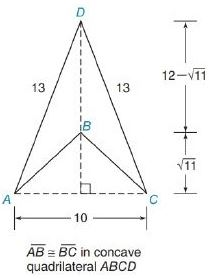Chapter 8.2, Problem 7EElementary Geometry For College St...

7th Edition
Alexander + 2 others
ISBN: 9781337614085

Solutions

Chapter
SectionElementary Geometry For College St...

7th Edition
Alexander + 2 others
ISBN: 9781337614085
Textbook Problem

In Exercises 1 to 8, find the perimeter of each polygon.To determine

To Find:

Perimeter of the quadrilateral provided.

Explanation

Formula Used:

The perimeter of a polygon is the sum of the lengths of all sides of the polygon.

Pythagorean theorem for the right angle triangle ABC for the hypotenuse AC,

AC2=AB2+BC2

Calculation:

As the two sides of the triangles ADC and ABC are equal in length, both the provided triangles are isosceles.

Let the altitude of the triangle touches the base AC at E. Since the provided triangles are isosceles, its altitude divides the base AC into two equal parts with respect to E.

That is, AE=EC=102=5

Still sussing out bartleby?

Check out a sample textbook solution.

See a sample solution

The Solution to Your Study Problems

Bartleby provides explanations to thousands of textbook problems written by our experts, many with advanced degrees!

Get Started

Find more solutions based on key concepts